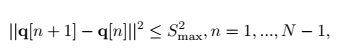# Are this constraint equal to this code?

code
` sum_square(Q_new(2:N,:)-Q(1:N-1,:),2) <= Smax^2 * ones(N-1,1) ;`Q_new is N * 2 matrix and Smax is scalar

Corrected version of post: Yes, I believe your code is correct.

help sum_square

sum_square Sum of squares.
For vectors, sum_square(X) is the sum of the squares of the elements of
the vector; i.e., SUM(X.^2).

``````For matrices, sum_square(X) is a row vector containing the application
of sum_square to each column. For N-D arrays, the sum_square operation
is applied to the first non-singleton dimension of X.

sum_square(X,DIM) takes the sum along the dimension DIM of X.

Disciplined convex programming information:
If X is real, then sum_square(X,...) is convex and nonmonotonic in
X. If X is complex, then sum_square(X,...) is neither convex nor
concave. Thus, when used in CVX expressions, X must be affine. DIM
must be constant.
``````

Here is a useful tip. Set Q to be a numerically populated matrix. Evaluate your candidate expression. if that is correct, it should also work when `Q` is a CVX variable.